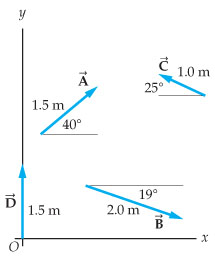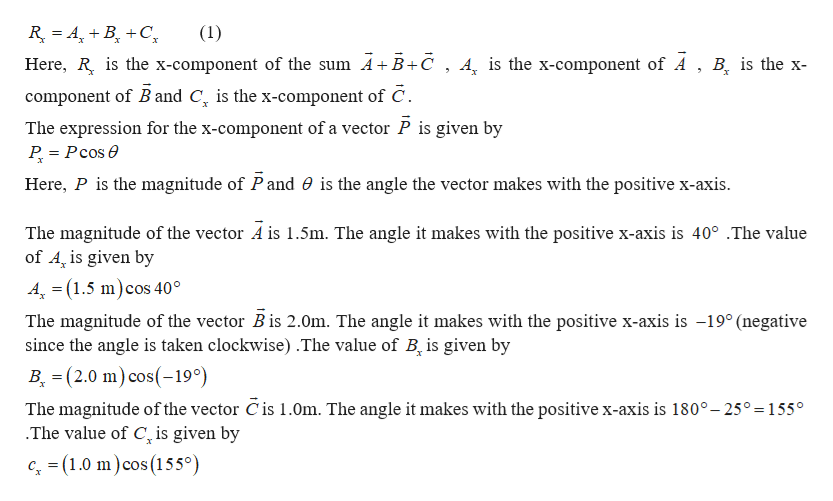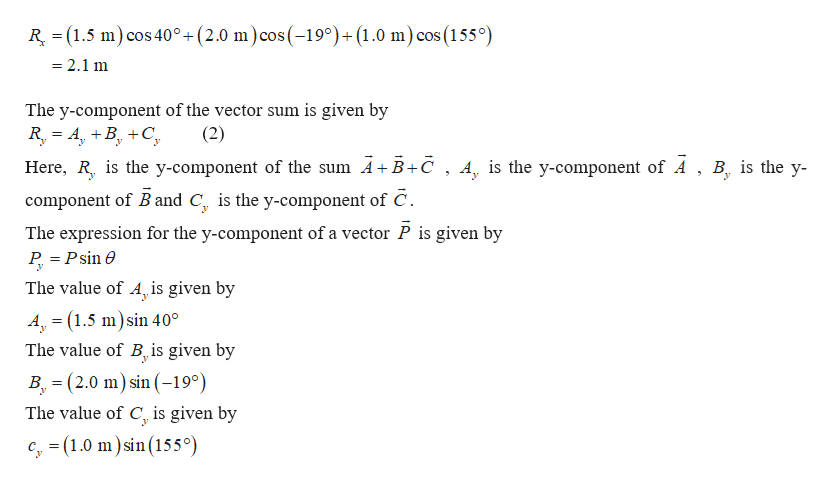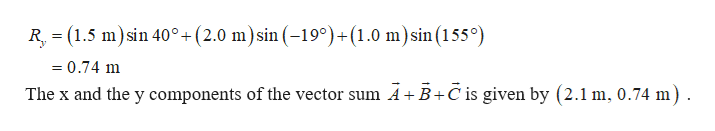Question
103 views
Referring to the vectors in the figure, express the sum A⃗ +B⃗ +C⃗  in unit vector notation.
(Figure 1)
Enter the x and y components of the vector separated by commahelp_outlineImage Transcriptionclosey 1.0 m A 25° 1.5 m 40° 19° 2.0 m B D 1.5 m fullscreen
check_circle

Step 1

The x-component of the vector sum is given byhelp_outlineImage Transcriptionclose(1) R ABC Here, R is the x-component of the sum A+ B+č , A, is the x-component of A , B is the x- component of B and C is the x-component of C The expression for the x-component of a vector P is given by P Pcos e Here, P is the magnitude of Pand 0 is the angle the vector makes with the positive x-axis The magnitude of the vector A is 1.5m. The angle it makes with the positive x-axis is 40° .The value of A, is given by 4 (1.5 m) cos 40° The magnitude of the vector Bis 2.0m. The angle it makes with the positive x-axis is -19° (negative since the angle is taken clockwise) .The value of B, is given by B (2.0 m) cos(19°) The magnitude ofthe vector C is 1.0m. The angle it makes with the positive x-axis is 180°-25° =155° .The value of C is given by c (1.0 m)cos (155°) fullscreen
Step 2

Put the above three equations in equation (1).help_outlineImage TranscriptioncloseR (1.5 m cos 40°+ (2.0 m)cos (-190)+(1.0 m) cos (155°) == = 2.1 m The y-component of the vector sum is given by R A,BC Here, R is the y-component of the sum A+ B+c , A, is the y-component of A , B is the y- component of B and C is the y-component of C (2) = The expression for the y-component of a vector P is given by P Psin e The value of A, is given by A (1.5 m)sin 400 The value of B is given by B, (2.0 m) sin (190) The value of C, is given by c, (1.0 m)sin (155°) fullscreen
Step 3

Put the above three equatio...help_outlineImage TranscriptioncloseR, (1.5 msin 40°+(2.0 m)sin (-19°)+(1.0 m)sin (155°) = 0.74 m The x and the y components of the vector sum AB+C is given by (2.1 m, 0.74 m) fullscreen

### Want to see the full answer?

See Solution

#### Want to see this answer and more?

Solutions are written by subject experts who are available 24/7. Questions are typically answered within 1 hour.*

See Solution
*Response times may vary by subject and question.
Tagged in

### Physics